Olympiad Test : Pattern

# Olympiad Test : Pattern

Test Description

## 20 Questions MCQ Test Maths Olympiad Class 6 | Olympiad Test : Pattern

Olympiad Test : Pattern for Class 6 2022 is part of Maths Olympiad Class 6 preparation. The Olympiad Test : Pattern questions and answers have been prepared according to the Class 6 exam syllabus.The Olympiad Test : Pattern MCQs are made for Class 6 2022 Exam. Find important definitions, questions, notes, meanings, examples, exercises, MCQs and online tests for Olympiad Test : Pattern below.
Solutions of Olympiad Test : Pattern questions in English are available as part of our Maths Olympiad Class 6 for Class 6 & Olympiad Test : Pattern solutions in Hindi for Maths Olympiad Class 6 course. Download more important topics, notes, lectures and mock test series for Class 6 Exam by signing up for free. Attempt Olympiad Test : Pattern | 20 questions in 40 minutes | Mock test for Class 6 preparation | Free important questions MCQ to study Maths Olympiad Class 6 for Class 6 Exam | Download free PDF with solutions
 1 Crore+ students have signed up on EduRev. Have you?
Olympiad Test : Pattern - Question 1

### Study the pattern and find the missing number.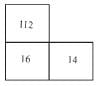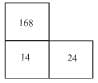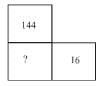Detailed Solution for Olympiad Test : Pattern - Question 1

From figure 1  (112 ÷ 14) × 2 = 16
From figure 2  (168 ÷ 24) × 2 = 14
From figure 3  (144 ÷ 16) × 2 = 9 × 2 = 18

Olympiad Test : Pattern - Question 2

### ​Study the pattern and find the missing number.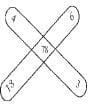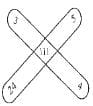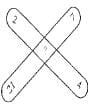Detailed Solution for Olympiad Test : Pattern - Question 2

From figure 1  4 × 6 + 18 × 3 = 24 + 54 = 78
From figure 2  3 × 5 + 24 × 4 = 15 + 96 = 111
From figure 3  2 × 7 + 21 × 4 = 14 + 84 = 98

Olympiad Test : Pattern - Question 3

### ​Study the pattern and find the missing number.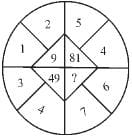Detailed Solution for Olympiad Test : Pattern - Question 3

From figure I 1 + 2 = 3 → 32 = 9
From figure II 3 + 4 = 7 → 72 = 49
5 + 4 = 9 → 92 = 81
7 + 6 = 13 →132 = 169

Olympiad Test : Pattern - Question 4

​​Study the pattern and find the missing number.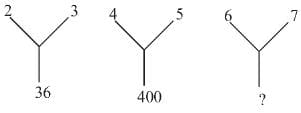Detailed Solution for Olympiad Test : Pattern - Question 4

From figure I    22 × 32 = 4 × 9 = 36
From figure II   42 × 52 = 16 × 25 = 400
From figure III  62 × 72 = 36 × 49 = 1764

Olympiad Test : Pattern - Question 5

​​​Study the pattern and find the missing number.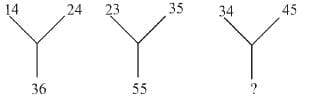Detailed Solution for Olympiad Test : Pattern - Question 5

From figure I   (14 + 24) – 2 = 38 – 2 = 36
From figure II  (23 + 35) – 3 = 58 – 3 = 55
From figure III (34 + 45) – 4 = 79 – 4 = 75

Olympiad Test : Pattern - Question 6

​Study the pattern and find the missing number.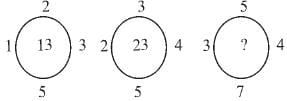Detailed Solution for Olympiad Test : Pattern - Question 6

From figure I   5 × 2 + 1 × 3 = 10 + 3 = 13
From figure II  2 × 4 + 5 × 3 = 8 + 15 = 23
From figure III 3 × 4 + 5 × 7 = 12 + 35 = 47

Olympiad Test : Pattern - Question 7

​​Study the pattern and find the missing number.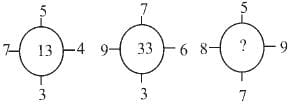Detailed Solution for Olympiad Test : Pattern - Question 7

From figure I   7 × 4 – 5 × 3 = 28 – 15 = 13
From figure II  9 × 6 – 7 × 3 = 54 – 21 = 33
From figure III 8 × 9 – 7 × 5 = 72 – 35 = 37

Olympiad Test : Pattern - Question 8

​​​Study the pattern and find the missing number.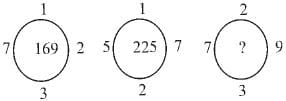Detailed Solution for Olympiad Test : Pattern - Question 8

From figure I  1 + 7 + 3 + 2 = 13 → 132 = 169
From figure II  1 + 5 + 2 + 7 = 15 → 152 = 225
From figure III  2 + 7 + 3 + 9 = 21 → 212 = 441

Olympiad Test : Pattern - Question 9

​​​​Study the pattern and find the missing number.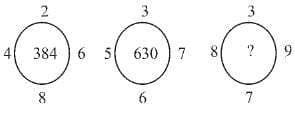Detailed Solution for Olympiad Test : Pattern - Question 9

2 × 4 × 8 × 6 = 384
3 × 5 × 6 × 7 = 630
3 × 8 × 7 × 9 = 1512

Olympiad Test : Pattern - Question 10

​​​​​Study the pattern and find the missing number.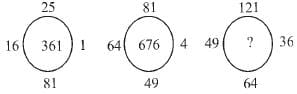Detailed Solution for Olympiad Test : Pattern - Question 10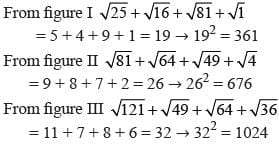Olympiad Test : Pattern - Question 11

​​​​​​Study the pattern and find the missing number.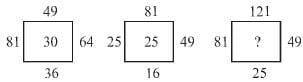Detailed Solution for Olympiad Test : Pattern - Question 11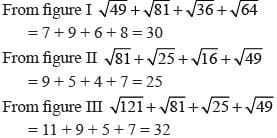Olympiad Test : Pattern - Question 12

​​​​​​​Study the pattern and find the missing number.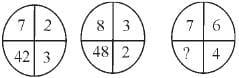Detailed Solution for Olympiad Test : Pattern - Question 12

From figure I   7 × 2 × 3 = 42
From figure II  8 × 3 × 2 = 48
From figure III 7 × 6 × 4 = 168

Olympiad Test : Pattern - Question 13

​​​​​​​​Study the pattern and find the missing number.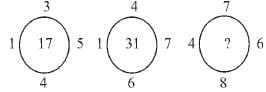Detailed Solution for Olympiad Test : Pattern - Question 13

From figure I 1 × 5 + 3 × 4 = 5 + 12 = 17
From figure II 1 × 7 + 4 × 6 = 7 + 24 = 31
From figure III 4 × 6 + 7 × 8 = 24 + 56 = 80

Olympiad Test : Pattern - Question 14

​​​​​​​​​Study the pattern and find the missing number.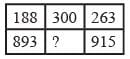Detailed Solution for Olympiad Test : Pattern - Question 14

In the first row, (263 – 188) × 4 = 300
In the second row, missing number
= (915 – 893) × 4 = 22 × 4 = 88

Olympiad Test : Pattern - Question 15

​​​​​​​​​​Study the pattern and find the missing number.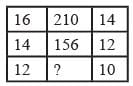Detailed Solution for Olympiad Test : Pattern - Question 15

In the first row, 16 × 14 – 14 = 210
In the second row, 14 × 12 – 12 = 156
∴ Missing number = 12 × 10 – 10 = 110

Olympiad Test : Pattern - Question 16

​​​​Study the pattern and find the missing number.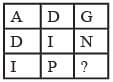Detailed Solution for Olympiad Test : Pattern - Question 16

If you observe there are two alphabets difference between ADG

Similarly, there are four alphabets difference between DIN

And, there are 6 alphabets difference between I and P, next alphabet will be the 7th alphabet after P.

A (2 alphabets) D (2 alphabets) G

D (4 alphabets) I (4 alphabets) N

I (6 alphabets) P (6 alphabets) W

So the answer is W

Olympiad Test : Pattern - Question 17

​​​​​​​​​​​​Study the pattern and find the missing number.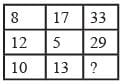Detailed Solution for Olympiad Test : Pattern - Question 17

In the first row, 8 × 2 + 17 = 33°,
In the second row; 12 × 2 + 5 = 29
∴ Missing number = 10 × 2 + 13 = 33

Olympiad Test : Pattern - Question 18

​​​​​​​​​​​​​Study the pattern and find the missing number.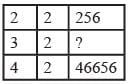Detailed Solution for Olympiad Test : Pattern - Question 18

In the first row, 2 + 2 = 4 and 44 = 256 In the third row, 4 + 2 = 6 and 66 = 46656
In the second row, 3 + 2 = 5
So, missing number = 55 = 3125

Olympiad Test : Pattern - Question 19

​​​​​​​​​​​​​​Study the pattern and find the missing number.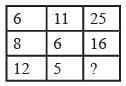Detailed Solution for Olympiad Test : Pattern - Question 19

In the first row, 11 × 2 + (6 ÷ 2) = 25
In the second row, 6 × 2 + (8 ÷ 2) = 16
∴ In the third row, missing number
= 5 × 2 + (12 ÷ 2) = 10 + 6 = 16

Olympiad Test : Pattern - Question 20

​​​​​​​​​​​​​​​Study the pattern and find the missing number.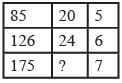Detailed Solution for Olympiad Test : Pattern - Question 20

In the first row, (85 ÷ 5) + 3 = 20
In the second row, (126 ÷ 6) + 3 = 24
∴ In the third row, missing number
= (175 ÷ 7) + 3
= (25 + 3) = 28

## Maths Olympiad Class 6

43 tests
 Use Code STAYHOME200 and get INR 200 additional OFF Use Coupon Code
Information about Olympiad Test : Pattern Page
In this test you can find the Exam questions for Olympiad Test : Pattern solved & explained in the simplest way possible. Besides giving Questions and answers for Olympiad Test : Pattern, EduRev gives you an ample number of Online tests for practice

43 tests